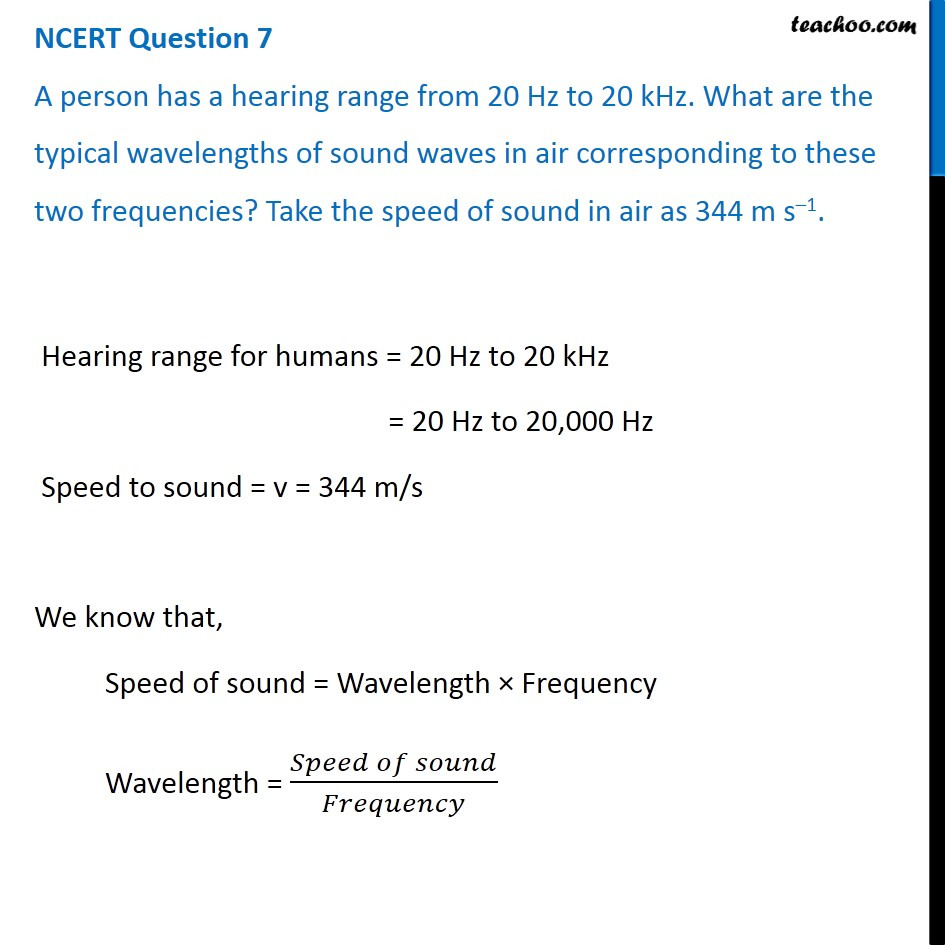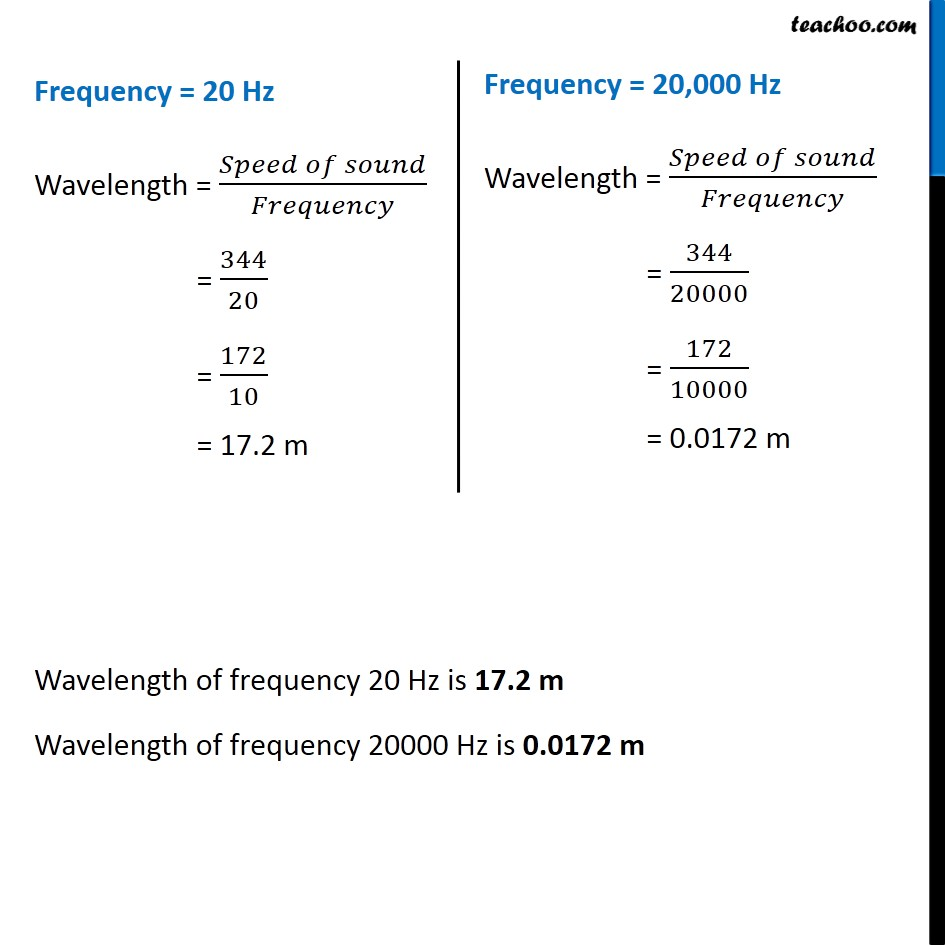NCERT Questions

Class 9
Chapter 12 Class 9 - SoundLearn in your speed, with individual attention - Teachoo Maths 1-on-1 Class

### Transcript

NCERT Question 7 A person has a hearing range from 20 Hz to 20 kHz. What are the typical wavelengths of sound waves in air corresponding to these two frequencies? Take the speed of sound in air as 344 m s–1. Hearing range for humans = 20 Hz to 20 kHz = 20 Hz to 20,000 Hz Speed to sound = v = 344 m/s We know that, Speed of sound = Wavelength × Frequency Wavelength = (𝑆𝑝𝑒𝑒𝑑 𝑜𝑓 𝑠𝑜𝑢𝑛𝑑)/𝐹𝑟𝑒𝑞𝑢𝑒𝑛𝑐𝑦 Frequency = 20 Hz Wavelength = (𝑆𝑝𝑒𝑒𝑑 𝑜𝑓 𝑠𝑜𝑢𝑛𝑑)/𝐹𝑟𝑒𝑞𝑢𝑒𝑛𝑐𝑦 = 344/20 = 172/10 = 17.2 m Frequency = 20,000 Hz Wavelength = (𝑆𝑝𝑒𝑒𝑑 𝑜𝑓 𝑠𝑜𝑢𝑛𝑑)/𝐹𝑟𝑒𝑞𝑢𝑒𝑛𝑐𝑦 = 344/20000 = 172/10000 = 0.0172 m Wavelength of frequency 20 Hz is 17.2 m Wavelength of frequency 20000 Hz is 0.0172 m